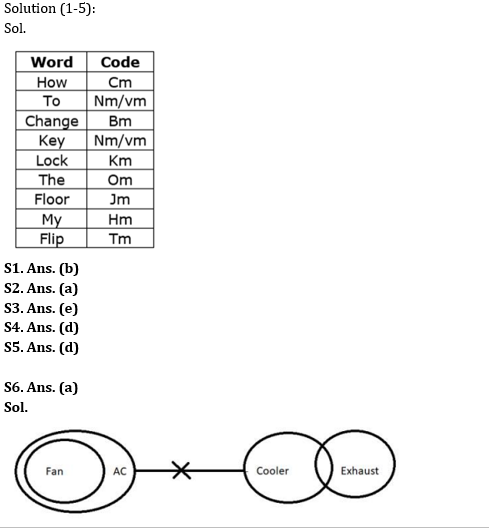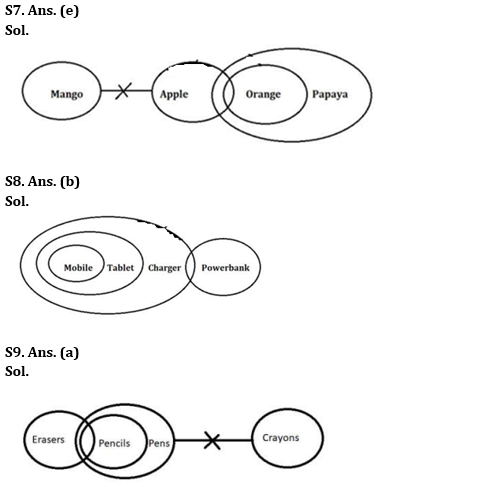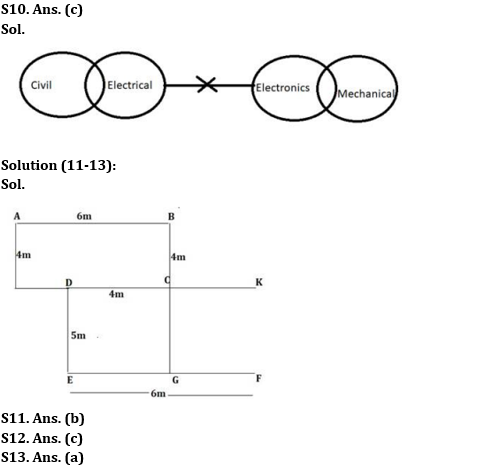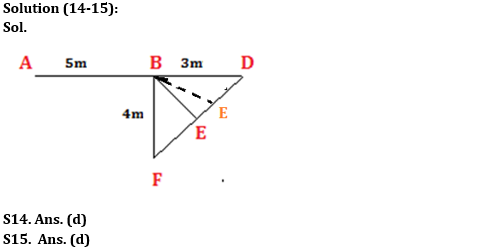Latest Banking jobs   »

# Reasoning Ability Quiz For SBI Clerk Prelims 2022- 17th May

Directions (1-5): Study the following information carefully and answer the following question.
In a code language,
‘How to change key’ is coded as ‘nm bm vm cm’
‘Lock the floor’ is coded as ‘om km jm’
‘Change my lock’ is coded as ‘bm km hm’
‘Floor flip how’ is coded as ‘jm cm tm’

Reasoning Ability Quiz For SBI Clerk Prelims 2022 CHECK IN HINDI

Q1. What is the code for ‘the’?
(a) km
(b) om
(c) jm
(d) cm
(e) Can’t be determined

Q2. What is the code for ‘Key to floor’?
(a) nm vm jm
(b) nm om jm
(c) bm vm cm
(d) km jm hm
(e) Can’t be determined

Q3. What is the code for ‘Key lock’?
(a) nm km
(b) cm km
(c) vm km
(d) jm hm
(e) Can’t be determined

Q4. Which words are coded as ‘hm cm km’?
(a) How to change
(b) How the lock
(c) The change to
(d) How my lock
(e) Can’t be determined

Q5. What could be the code for ‘Change far flip’ if ‘far’ is coded as ‘pm’?
(a) hm jm tm
(b) om jm cm
(c) km jm hm
(d) bm tm pm
(e) bm nm tm

Directions (6-10): In the question below are given three statements followed by two conclusions. You have to take the given statements to be true even if they seem to be at variance with commonly known facts. Read all the conclusions and then decide which of the given conclusions logically follows from the given statements disregarding commonly known facts.

Q6. Statements: All Fans are AC. No AC is Cooler. Some Coolers are Exhaust.
Conclusions:
I. Some AC being Exhaust is a possibility.
II. All ACs are Fan
(a) Only I follows
(b) Only II follows
(c) Either I or II follows
(d) Neither I nor II follows
(e) Both I and II follow

Q7. Statements: No Mango is Apple. Only a few Apple are Orange. All Oranges are Papaya.
Conclusions:
I. Some Papayas are Apples
II. Some Apples are not Oranges.
(a) Only I follows
(b) Only II follows
(c) Either I or II follows
(d) Neither I nor II follows
(e) Both I and II follow

Q8. Statements: All Mobiles are Tablets. All Tablets are Chargers. Only a few Chargers are Powerbanks.
Conclusions:
I. Some Powerbanks are Tablets.
II. Some Chargers are Mobile.
(a) Only I follows
(b) Only II follows
(c) Either I or II follows
(d) Neither I nor II follows
(e) Both I and II follow

Q9. Statements: Some Erasers are Pencils. All Pencils are Pens. No Pens are Crayons.
Conclusions:
I. Some Erasers can be Crayons.
II. No Erasers is Crayons.
(a) Only I follows
(b) Only II follows
(c) Either I or II follows
(d) Neither I nor II follows
(e) Both I and II follow

Q10. Statements: Only a few Civil are Electrical.No Electrical is Electronics. Only a few Electronics are Mechanical.
Conclusions:
I. Some Mechanical is not Electronics
II. All Mechanical is Electronics.
(a) Only I follows
(b) Only II follows
(c) Either I or II follows
(d) Neither I nor II follows
(e) Both I and II follow

Directions (11-13): Answer the question based on the information given below.
Point A is 6m to the west of point B. Point D is 4m to the west of point C. Point G is 9 to the south of point B. Point C is 5m to the north of point G. Point E is 6m to the west of point F. Point D is 5m to the north of point E.

Q11. What is the distance between point C and point B?
(a) 5m
(b) 4m
(c) 3m
(d) 2m
(e) 6m

Q12. If a person is standing at point A and then he walks towards his south and walks for 4m then he takes a left turn and walks for 8m and stopped at point K then point K is in which of the following direction with respect to point G?
(a) North-west
(b) South-west
(c) North-east
(d) South-east
(e) South

Q13. What is the distance between point F and point G?
(a) 2m
(b) 3m
(c) 5m
(d) 4m
(e) 6m

Directions (14-15): Study the information given below and answer the questions based on it.
There are five persons A, B, F, D and E sitting randomly. F is to the southwest of D. B is 3m to the west of D who is 8m to the east of A. E who is to the southeast of B is on the line FD where F is 4m to the south of B.

Q14. In which direction is E with respect to A?
(a) East
(b) South
(c) South-west
(d) South – east
(e) None of these

Q15. What is the distance between B and E?
(a) 3m
(b) 4m
(c) 5m
(d) Can’t be determined
(e) None of these

Solutions#### Congratulations!